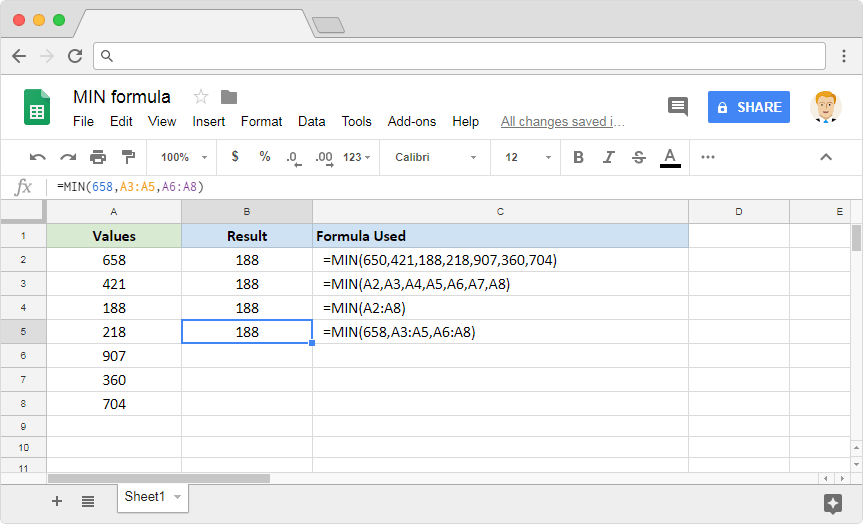# How to use the MIN formula in Google SheetsWhile the first parameter accepts only range references, the second parameter can take both direct numbers as well as cell references.

We have the input dataset laid out on column A, which will serve as the source for the first parameter. We have placed the same dataset on column B, except we sorted it in the descending order. Column B is just for reference so that we can quickly compare the outputs with the expected output.

Now let us quickly go through the column D. On the cell D2, we are looking to extract the first largest value. On D3, the second largest value and so on and so forth. If we were to compare columns B and D, we will notice that the LARGE formula returns the outputs the exact manner that it is expected to do so.The first example consumes direct numeric values and returns the minimum value out of them. The second example is a demonstration that shows us that the MIN formula can use references to cells that store numbers. In the third example, instead of sending multiple values or references, we have passed a single composite range. This composite cell range consists of all the values from A2 through A8. And, in the final example, we not only passed direct numeric values but also a couple range references.

You’ll observe that the output is the same across all examples, regardless of the approach that we have taken with the formula. And unsurprisingly, if we look closely, 188 is the least value among all the numbers we used for input parameters. So, the MIN formula was spot on!

The MAX formula in Google Sheets identifies the maximum value from a set of numeric values. Read all about it in this blog post.